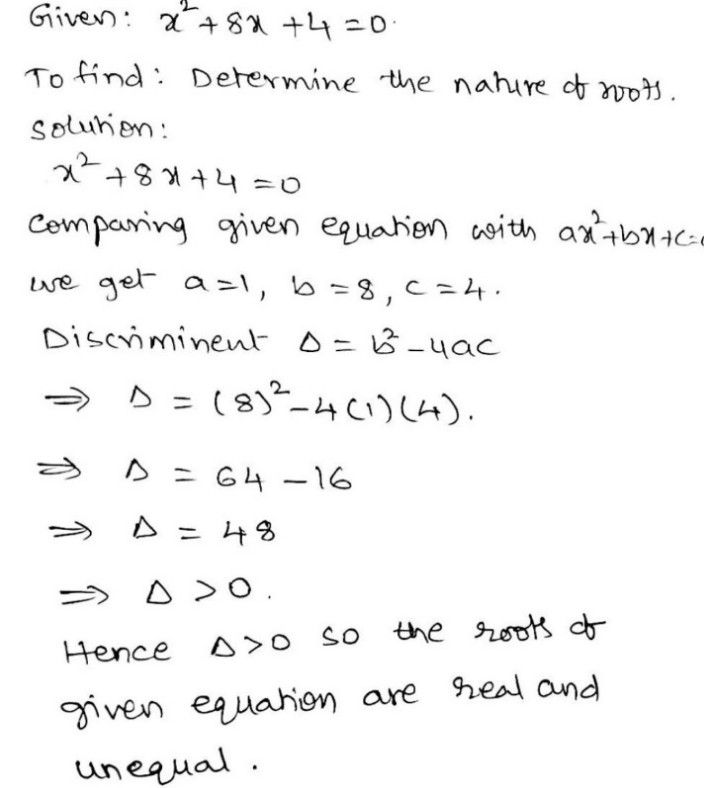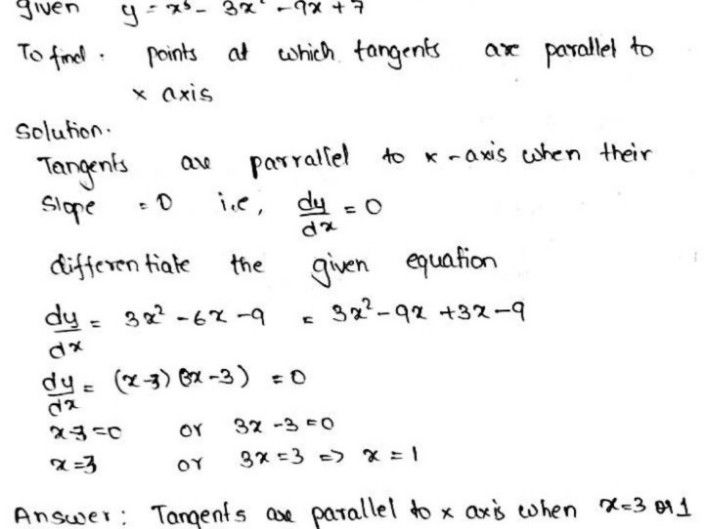Symbol
ProblemLearning Task 2. Sketch the graph of the following. Give the domain and range of the following quadratic function defined by the given equation. Determine the direction where the parabola opens, its vertex, its axis of symmetry, and its x- and y- intercepts. $1.y=x^{2+8x+4}$ $3.y=-3x^{2+12x-7}$ - $y=x2+4x+5$ $4.y=2x^{2-4x-6}$
7th-9th grade
Algebra
Search count: 111
SolutionQanda teacher - manoj